# Algebraic Expressions (Basics)

##By Anywhere Math# Writing Expressions

##By Anywhere Math##By Anywhere Math# Adding and Subtracting Linear Expressions

##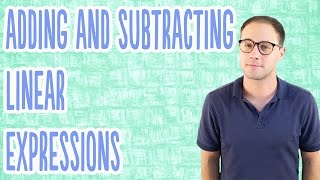By Anywhere Math# Algebra Basics: The Distributive Property - Math Antics

##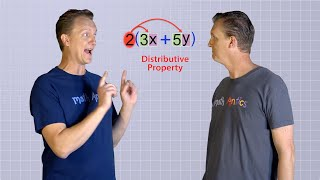By mathantics# Solving Inequalities with Addition or Subtraction

##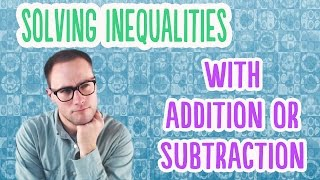By Anywhere Math# Properties of Addition and Multiplication

##By Anywhere Math# How To Integrate Using U-Substitution

##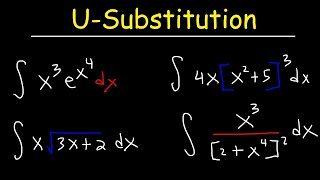By The Organic Chemistry Tutor

This calculus video tutorial provides a basic introduction into u-substitution. It explains how to integrate using u-substitution. You need to determine which part of the function to set equal to the u variable and you to find the derivative of u to get du and solve for dx. After replacing all x variables with u variables, find the antiderivative of f(u) and substitute u in the new function with x variables. This video contains plenty of examples and practice problems of finding the indefinite integral using u-substitution. Examples include polynomial functions, trigonometric functions, exponential functions, square root functions, and rational functions.# The Distributive Property

##By Anywhere Math# High School Math

##By

High school math lesson.# Trigonometric Substitution

##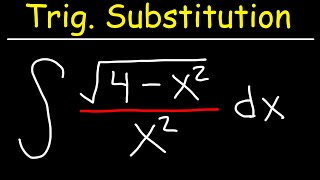By The Organic Chemistry Tutor

This calculus video tutorial provides a basic introduction into trigonometric substitution. It explains when to substitute x with sin, cos, or sec. It also explains how to perform a change of variables using u-substitution integration techniques and how to use right triangle trigonometry with sohcahtoa to convert back from angles in the form of theta to an x variable. There's plenty of examples and practice problems in this lesson.# Writing simple algebric expressions

##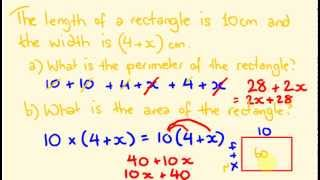By tecmath# Integration By Partial Fractions

##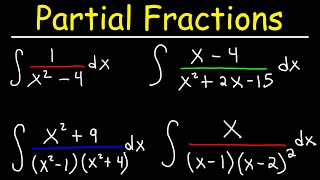By The Organic Chemistry Tutor

This calculus video tutorial provides a basic introduction into integrating rational functions using the partial fraction decomposition method. Partial fraction decomposition is the process of breaking a single complex fraction into multiple simpler fractions. The integrals of many rational functions lead to a natural log function with absolute value expressions. This video explains what to do when you have repeated linear factors and quadratic factors. This tutorial contains many examples and practice problems on integration by partial fractions.# Antiderivatives

##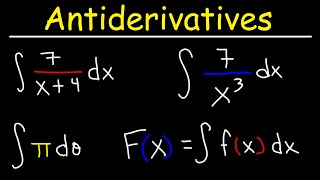By The Organic Chemistry Tutor

This calculus video tutorial provides a basic introduction into antiderivatives. It explains how to find the indefinite integral of polynomial functions as well as rational functions.# 08 - Solving Exponential Equations - Part 1 - Solve for the Exponent

##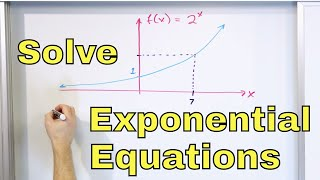By Math and Science

Quality Math And Science Videos that feature step-by-step example problems!# ALL OF GRADE 9 MATH IN 60 MINUTES!!! (exam review part 1)

##By Lumos Learning

Here is a great exam review video reviewing all of the main concepts you would have learned in the MPM1D grade 9 academic math course. The video is divided in to 3 parts. This is part 1: Algebra. The main topics in this section are exponent laws, polynomials, distributive property, and solving first degree equations. Please watch part 2 and 3 for a review of linear relations and geometry. If you watch all 3 parts, you will have reviewed all of grade 9 math in 60 minutes. Enjoy! Visit jensenmath.ca for more videos and course materials.# 06 - Proving the Logarithm (Log) Rules - Understand Logarithm Rules & Laws of Logs

##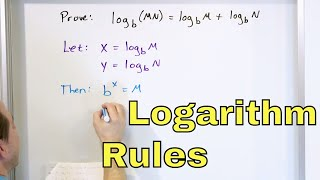By Math and Science

Quality Math And Science Videos that feature step-by-step example problems!# Writing Equations of Ellipses In Standard Form and Graphing Ellipses - Conic Sections

##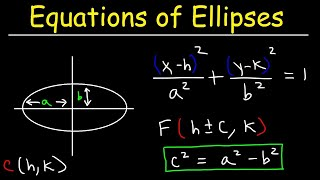By The Organic Chemistry Tutor

This algebra video tutorial explains how to write the equation of an ellipse in standard form as well as how to graph the ellipse when in standard form. It explains how to find the coordinates of the foci, vertices, and co-vertices. This video contains plenty of examples and practice problems.# 10 - What are Imaginary Numbers?

##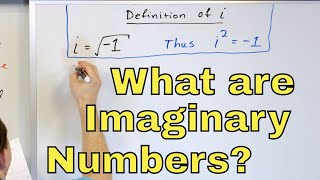By Math and Science

Quality Math And Science Videos that feature step-by-step example problems!# Algebra Introduction - the basics

##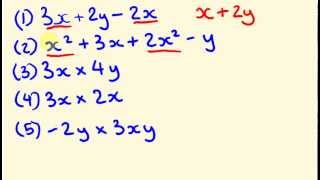By tecmath